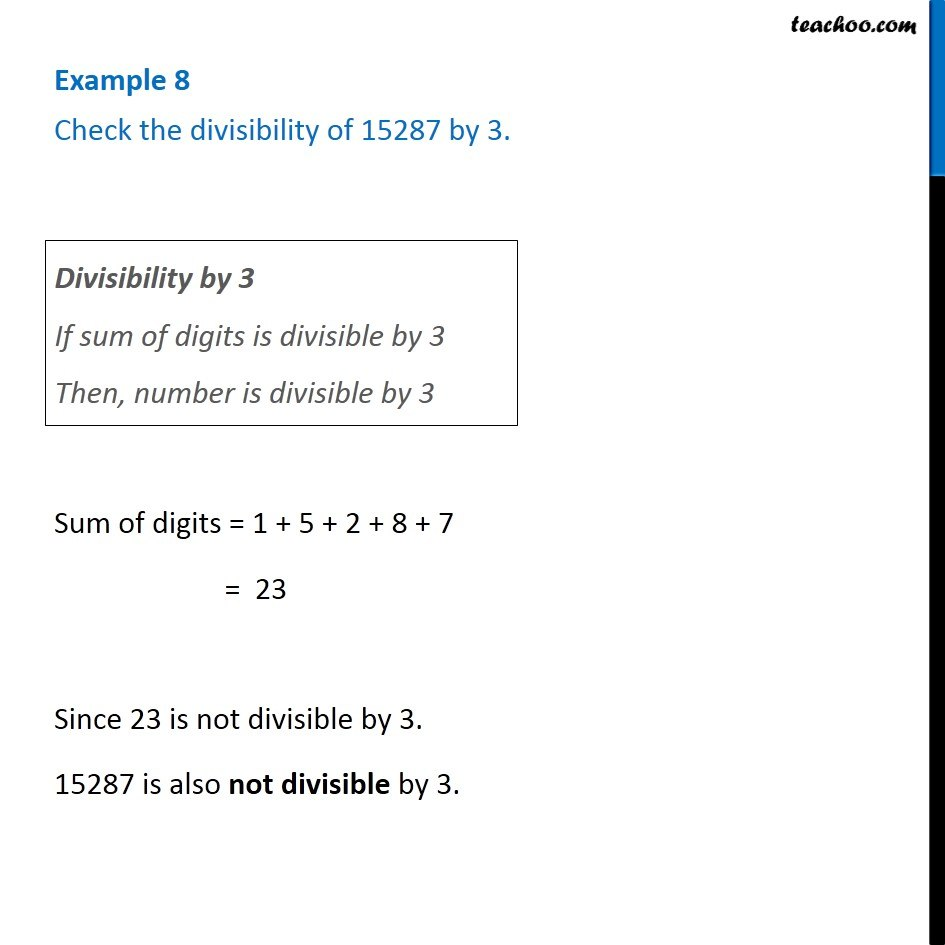Examples

Chapter 16 Class 8 Playing with Numbers
Serial order wiseGet live Maths 1-on-1 Classs - Class 6 to 12

### Transcript

Example 8 Check the divisibility of 15287 by 3. Divisibility by 3 If sum of digits is divisible by 3 Then, number is divisible by 3 Sum of digits = 1 + 5 + 2 + 8 + 7 = 23 Since 23 is not divisible by 3. 15287 is also not divisible by 3.